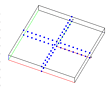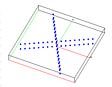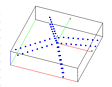Example 1: test using rotatept to modify a point distribution.

The objective is to use the rotatept command to modify a point distribution by rotation.

The output consists of three gmv files, one showing the original point distribution, one showing a rotation in the xy-plane, and one showing a rotation away from the z-axis, each using rotatept/rtz.

Input

lagrit_input_rotatept

Images of GMV input and output

Initial point distributionrotation in xy-planedrotation in xy-plane and away from z-axis